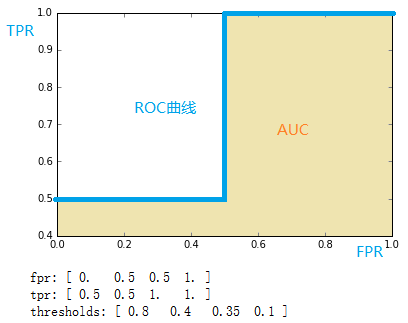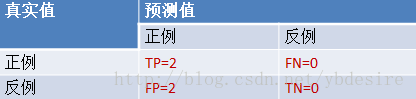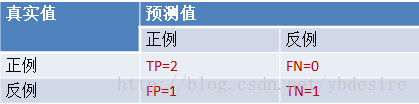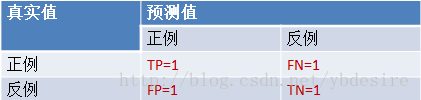# ROC与AUC的定义与使用详解

Precision精准度from sklearn.metrics import precision_score
Recall召回率from sklearn.metrics import recall_score
F1F1值from sklearn.metrics import f1_score
Confusion Matrix混淆矩阵from sklearn.metrics import confusion_matrix
ROCROC曲线from sklearn.metrics import roc
AUCROC曲线下的面积from sklearn.metrics import auc

Mean Square Error (MSE, RMSE)平均方差from sklearn.metrics import mean_squared_error
Absolute Error (MAE, RAE)绝对误差from sklearn.metrics import mean_absolute_error, median_absolute_error
R-SquaredR平方值from sklearn.metrics import r2_score

ROC和AUC定义
ROC全称是“受试者工作特征”（Receiver Operating Characteristic）。ROC曲线的面积就是AUC（Area Under the Curve）。AUC用于衡量“二分类问题”机器学习算法性能（泛化能力）。

`截断点`取不同的值，`TPR``FPR`的计算结果也不同。将`截断点`不同取值下对应的`TPR``FPR`结果画于二维坐标系中得到的曲线，就是ROC曲线。横轴用FPR表示。

sklearn计算ROC
sklearn给出了一个计算ROC的例子。

```y = np.array([1, 1, 2, 2])
scores = np.array([0.1, 0.4, 0.35, 0.8])
fpr, tpr, thresholds = metrics.roc_curve(y, scores, pos_label=2)```

``````fpr = array([ 0. ,  0.5,  0.5,  1. ])
tpr = array([ 0.5,  0.5,  1. ,  1. ])
thresholds = array([ 0.8 ,  0.4 ,  0.35,  0.1 ])#截断点`````````y = np.array([1, 1, 2, 2])
scores = np.array([0.1, 0.4, 0.35, 0.8])```

1. 分析数据
y是一个一维数组（样本的真实分类）。数组值表示类别（一共有两类，1和2）。我们假设y中的1表示反例，2表示正例。即将y重写为：

`y_true = [0, 0, 1, 1]`

score即各个样本属于正例的概率。

2. 针对score，将数据排序

y0.1N
y0.35P
y0.4N
y0.8P

`截断点`依次取值为0.1,0.35,0.4,0.8时，计算`TPR``FPR`的结果。

``````scores = [0.1, 0.4, 0.35, 0.8]
y_true = [0, 0, 1, 1]
y_pred = [1, 1, 1, 1]``````TPR = TP/(TP+FN) = 1
FPR = FP/(TN+FP) = 1

```scores = [0.1, 0.4, 0.35, 0.8]
y_true = [0, 0, 1, 1]
y_pred = [0, 1, 1, 1]```TPR = TP/(TP+FN) = 1
FPR = FP/(TN+FP) = 0.5

```scores = [0.1, 0.4, 0.35, 0.8]
y_true = [0, 0, 1, 1]
y_pred = [0, 1, 0, 1]```TPR = TP/(TP+FN) = 0.5
FPR = FP/(TN+FP) = 0.5

```scores = [0.1, 0.4, 0.35, 0.8]
y_true = [0, 0, 1, 1]
y_pred = [0, 0, 0, 1]```TPR = TP/(TP+FN) = 0.5
FPR = FP/(TN+FP) = 0

• TPR：真实的正例中，被预测正确的比例
• FPR：真实的反例中，被预测正确的比例

1. <ahref="http://scikit-learn.org/stable/modules/generated/sklearn.metrics.roc_curve.html" rel="nofollow">http://scikit-learn.org/stable/modules/generated/sklearn.metrics.roc_curve.html
2. ROC计算公式，http://blog.yhat.com/posts/roc-curves.html
3. 《机器学习》，周志华

• 本文由 发表
• 转载请务必保留本文链接：https://www.plob.org/article/22905.html